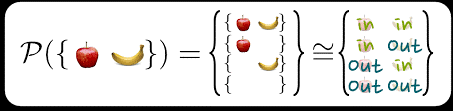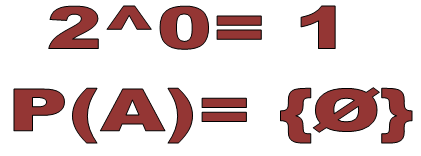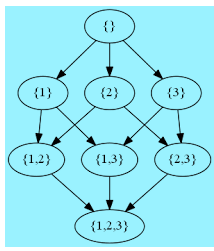×#### Thank you for registering.

One of our academic counsellors will contact you within 1 working day.

Click to Chat

1800-1023-196

+91-120-4616500

CART 0

• 0

MY CART (5)

Use Coupon: CART20 and get 20% off on all online Study Material

ITEM
DETAILS
MRP
DISCOUNT
FINAL PRICE
Total Price: Rs.

There are no items in this cart.
Continue Shopping```Power Set

Table of Content

Definition of Power Set

Cardinality of Power Set

Power Set proof

Power Set of Empty Set

What is the Power Set of a set having Empty Set as an element?

Power Set of a Power Set

Power Sets and Partially ordered Sets

Power Set and Lattices

Power Set Algorithm

Definition of Power Set

A set is a collection of different objects having some common property. These objects are the elements of the set. The elements have their own identity separately so all the elements make the subsets of the set.

And the set of all the subsets is the power set of that particular set, including null set and itself also.

If A is the set then the set of all the subsets of A is the power set of A. The power set of A will be represented by P(A).Example

Z = {1, 3, 5}

Here, the number of the elements of Z is 3.

The subsets of Z are { }, {1}, {3}, {5}, {1, 3}, {3, 5}, {1, 5}, {1, 3, 5}

The power set of Z is the set of all the subsets of Z.We will list all the subsets then enclose them in the curly braces “{ }”

P(Z) = { { }, {1}, {3}, {5}, {1, 3}, {3, 5}, {1, 5}, {1, 3, 5} }

Example

Here the two elements of the set are apple and banana.

So the subsets of it could be empty set, apple, banana,apple and banana both.

The power set of this set will be the set of all the subsets as shown above in picture.Cardinality of Power Set

Cardinality is the number of elements of a set.The number of elements of a power set is the number of subsets.The number of elements is represented by |Z|. As we know that the formula of calculating number of subsets is 2n so the number of elements of a power set will also be 2n as the power set is the set of all the subsets of a set.

We can write it as:

|P(Z)| = 2n

Example

Z = {1, 2, 3}

n(Z) = 3 i.e. the number of elements of Z is 3.The subsets of Z are { },{1},{2},{3},{1,2},{2,3},{1,3},{1,2,3}

|P(Z)| = 2n =23= 8

P(Z)={ { }, {1}, {2}, {3}, {1, 2}, {2, 3}, {1, 3}, {1, 2, 3} } i.e. a set of all the subsets of Z.

Power Set proof

Now we need to prove that|P(Z)| = 2n is correct for all finite sets.

We will Proof by induction

For all n∈N,

|S| =n ⟹ |P(S)| = 2n

As we know that the cardinality of empty set is zero:

S = ∅ ⟺ |S| = 0

Then:

P(∅) = {∅}

The power set of an empty set has one element, that is, ∅.

So:

|P(∅)| = |{∅}| = 1 = 20

Induction Hypothesis

Now we need to show that, if P(z) is true, where z≥2, then it logically follows that P(z+1) is also true.

So here is our induction hypothesis:

|S| = z ⟹ |P(S)| =2z

Now we need to prove that-

|S| = z+1 ⟹ |P(S)| = 2z+1

Induction Step

Let |S| = z+1.

Let y∈S. i.e. y is element of S.

Consider the set T = S-{y} where y is any element of S but not of T.

We see that |T| = z.

Now adjoin y also to all the subsets of T

Now while counting the subsets of S , we have:

all the subsets of T with y adjoined to them.

From the induction hypothesis, there are 2z subsets of T.

Adding y to each of these does not change their number, so there are another 2z subsets of S consisting of all the subsets of T with y adjoined to them.

In total that makes 2z+2z

=2×2z

=2z+1 subsets of S.

So P(z) ⟹ P(z+1) and the result follows by the Principle of Mathematical Induction.

Therefore:

∀n∈N:|S| = n ⟹ |P(S)| = 2n

Power Set of Empty Set

The empty set is the set having no or zero element. Its cardinality is zero(0).It is also called null or void set.

The power set is the set of all the subsets of a set. We know that every set is a subset of itself and empty set is also the subset of itself.

So the set containing only the empty set is the power set of an empty set.

If A is the empty set i.e. A = ∅

P(A) = {∅}This is a singleton set as the set having only one element is called the singleton set.

The important point is that the empty set is the set with zero element but the power set of an empty set is not empty set because the power set of an empty set contains one element that is ∅.

What is the Power Set of a set having Empty Set as an element?

First,we need to understand the difference between the empty set and the set with the empty set as an element. In the empty set, we don’t have any element i.e. the zero cardinality but if we have a set with “∅” as the element, then this shows that it has one element.

Example

Z= {}, i.e. Z is the empty set having zero element.

Y= {∅}, i.e. Y is a set with one element i.e. ∅.

Here the power set of Z i.e. empty set is {∅}

P (Z) = {∅}

But the power set of Y=21=2

Subsets of Y are ∅ as empty set is subset of every set and {∅} as the element of set Y.So the power set of Y is

P(Y) = {∅,{∅}  }

Power Set of a Power Set

Aswe know that the power set is the set of all the subsets of any given set then what about the power set of a power set?Let’s understand it with an example-

M = {a}, |M|=1, i.e. the number of elements of M is 1. So the number of elements of power set of M will be 21= 2.

P(M) = { { },{a} }

The subsets of P(M) are { },

{{ }},

{{a}},

{ { },{a}}

|P(P(M))| = 22 = 4

P(P(M)) = { { }, {{ }}, {{a}}, { { }, {a}}}

And if we want to calculate the power set of the power set of the power set of M i.e. P(P(P(M))), again then we can do it with the same process again as done above.

Power Sets and Partially ordered Sets

All the elements of a powerset have some natural ordering, ⊆, and any power set and ⊆ form a set ordered. A set is an ordered set if their elements have an order, and a partially ordered set is said so because it’s all pairs of elements are not ordered.

Example

P({x, y}) = {∅, {y}, {x}, {x,y} }

Some pairs of elements of P({x,y}) are ordered,

Like,

∅ ⊆ {y} and {y} ⊆ {x,y}.  But {x} and {y} are not ordered by ⊆, because neither {x} ⊆ {y} nor {y} ⊆ {x}.

It meansP({x,y}) is a partially ordered set.

Power Set and Lattices

If P be an ordered set.  P is a lattice  if for every pair x,y∈P, their LUB (least upper bound)  x∨y and GLB (greatest lower bound) x∧y are both elements of P.

The LUB is the union of the two elements.

The GLB is their intersection.

Another way of saying the same thing:  an ordered set P is a lattice if it is closed under the cap and cup operations.

A lattice may have a top and/or a bottom, but an infinite lattice need not have either. A finite lattice always has a top and a bottom.This is the lattice of a power set of P{1, 2, 3}

This shows the elements of the power set of {1, 2, 3}

Subsets with 0 elements- { }

Subsets with 1 element- {1}, {2}, {3}

Subsets with 2 elements- {1, 2}, {2, 3}, {1, 3}

Subsets with 3 elements- {1, 2, 3}

A power set is a lattice as-

It is a partially ordered set, and

each pair of elements has a least upper bound (LUB) and a greatest lower bound (GLB).

LUB

The union of two elements is an upper bound for them, because for any two sets S and T, S⊆S∪T.

The union of two elements is the least upper bound for S and T because there is no set R smaller than S∪T that contains both S and T.

GLB

The intersection of two elements is the lowest bound for them as for any two sets S and T, { }⊆S∩T

The intersection of two elements is the greatest lower bound for S and T because there is no set R greater than S∩T that does not contain empty set.

Power Set Algorithm

For any set S, if it is a finite set, there is a recursive algorithm to calculate P(S).

The recursive algorithm is the term of computer science.The recursion is the way of defining an infinite set of objects by a finite statement.

The power set algorithm lies on the binary representation of increasing number to construct subsets.

The elements of the power set of a set of n elements match to the n-bit binary integers from 0 to (2n-1):

Thus, we can itemize the elements of a power set by counting from 0 to (2n-1) and for each number giving the subset having the elements related to the 1 bit.

We need to write down the integer’s variable and the sequence of binary numbers then decode each integer as binary {000, 001, 010, ... } and take 1 as an element present and 0 as an element absent.

If A = {x, y, z}

We will follow the following algorithm:

abc
Subset

0
0
{ }

1
1
{z}

2
10
{y}

3
11
{y,z}

4
100
{x}

5
101
{x,z}

6
110
{x,y}

7
111
{x,y,z}

Here n(A) = 3 According to formula P(A) = 23 =8

And in algorithm we have to count from 0 to (2n-1).

(2n-1) = 23-1 = 7

So, we had count from 0 to seven.

Here the first set is empty set and the last set has all the elements in it.

Power Set
```### Course Features

• 731 Video Lectures
• Revision Notes
• Previous Year Papers
• Mind Map
• Study Planner
• NCERT Solutions
• Discussion Forum
• Test paper with Video Solution